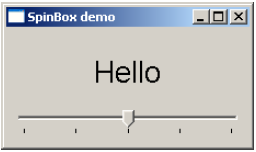# PyQt - QSlider Widget & Signal

QSlider class object presents the user with a groove over which a handle can be moved. It is a classic widget to control a bounded value. Position of the handle on the groove is equivalent to an integer between the lower and the upper bounds of the control.

A slider control can be displayed in horizontal or vertical manner by mentioning the orientation in the constructor.

```self.sp = QSlider(Qt.Horizontal)
self.sp = QSlider(Qt.Vertical)
```

The following table lists some of the frequently used methods of QSlider class −

Given below are the most commonly used methods of QSlider.

Sr.No. Methods & Description
1

setMinimum()

Sets the lower bound of the slider

2

setMaximum()

Sets the upper bound of the slider

3

setSingleStep()

Sets the increment/decrement step

4

setValue()

Sets the value of the control programmatically

5

value()

Returns the current value

6

setTickInterval()

Puts the number of ticks on the groove

7

setTickPosition()

Places the ticks on the groove. Values are −

 QSlider.NoTicks No tick marks QSlider.TicksBothSides Tick marks on both sides QSlider.TicksAbove Tick marks above the slider QSlider.TicksBelow Tick marks below the slider QSlider.TicksLeft Tick marks to the left of the slider QSlider.TicksRight Tick marks to the right of the slider

## QSlider Signals

Sr.No. Methods & Description
1

valueChanged()

When the slider's value has changed

2

sliderPressed()

When the user starts to drag the slider

3

sliderMoved()

When the user drags the slider

4

sliderReleased()

When the user releases the slider

valueChanged() signal is the one which is most frequently used.

### Example

The following example demonstrates the above functionality. A Label and a horizontal slider is placed in a vertical layout. Slider’s valueChanged() signal is connected to valuechange() method.

```self.sl.valueChanged.connect(self.valuechange)
```

The slot function valuechange() reads current value of the slider and uses it as the size of font for label’s caption.

```size = self.sl.value()
self.l1.setFont(QFont("Arial",size))
```

The complete code is as follows −

```import sys
from PyQt4.QtCore import *
from PyQt4.QtGui import *

class sliderdemo(QWidget):
def __init__(self, parent = None):
super(sliderdemo, self).__init__(parent)

layout = QVBoxLayout()
self.l1 = QLabel("Hello")
self.l1.setAlignment(Qt.AlignCenter)

self.sl = QSlider(Qt.Horizontal)
self.sl.setMinimum(10)
self.sl.setMaximum(30)
self.sl.setValue(20)
self.sl.setTickPosition(QSlider.TicksBelow)
self.sl.setTickInterval(5)

self.sl.valueChanged.connect(self.valuechange)
self.setLayout(layout)
self.setWindowTitle("SpinBox demo")

def valuechange(self):
size = self.sl.value()
self.l1.setFont(QFont("Arial",size))

def main():
app = QApplication(sys.argv)
ex = sliderdemo()
ex.show()
sys.exit(app.exec_())

if __name__ == '__main__':
main()
```

The above code produces the following output −The font size of the label changes as handle of the slider is moved across the handle.